Updating search results...

# 39 Results

View
Selected filters:
• NC.Math.8.NS.1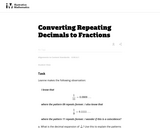Unrestricted Use
CC BY
Rating
0.0 stars

This is a task from the Illustrative Mathematics website that is one part of a complete illustration of the standard to which it is aligned. Each task has at least one solution and some commentary that addresses important asects of the task and its potential use. Here are the first few lines of the commentary for this task: Leanne makes the following observation: I know that \frac{1}{11} = 0.0909\ldots where the pattern 09 repeats forever. I also know that \frac{1}{9} = 0....

Subject:
Mathematics
Material Type:
Activity/Lab
Provider:
Illustrative Mathematics
Provider Set:
Illustrative Mathematics
Author:
Illustrative Mathematics
10/14/2013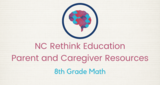Conditional Remix & Share Permitted
CC BY-NC-SA
Rating
0.0 stars

This 8th grade Math&nbsp;parent guide&nbsp;explains the content in straightforward terms so they can support their children&rsquo;s learning at home and will&nbsp;encourage&nbsp;caretaker engagement&nbsp;with lessons.

Subject:
Mathematics
Material Type:
Reference Material
Author:
Kelly Rawlston
Letoria Lewis
01/10/2022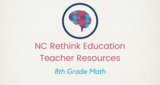Conditional Remix & Share Permitted
CC BY-NC-SA
Rating
0.0 stars

Our Teacher Guides are meant to support the use of our online course and unit content. Please use these to accompany the use of our content and for ideas to support struggling learners, those needing extension and for additional resources.

Subject:
Mathematics
Material Type:
Curriculum
Teaching/Learning Strategy
Author:
Kelly Rawlston
Letoria Lewis
05/16/2022Conditional Remix & Share Permitted
CC BY-NC
Rating
0.0 stars

In CK-12 Middle School Math Concepts Grade 8, the learning content is divided into concepts. Each concept is complete and whole providing focused learning on an indicated objective. Theme-based concepts provide students with experiences that integrate the content of each concept. Students are given opportunities to practice the skills of each concept through real-world situations, examples, guided practice and explore more practice. There are also video links provided to give students an audio/visual way of connecting with the content.

Subject:
Mathematics
Material Type:
Full Course
Lesson Plan
Textbook
Unit of Study
Provider:
CK-12 Foundation
Provider Set:
CK-12 FlexBook
12/06/2018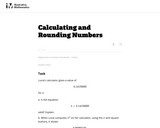Unrestricted Use
CC BY
Rating
0.0 stars

This task is intended for instructional (rather than assessment) purposes, providing an opportunity to discuss technology as it relates to irrational numbers and calculations in general. The task gives a concrete example where rounding and then multiplying does not yield the same answer as multiplying and then rounding.

Subject:
Mathematics
Material Type:
Activity/Lab
Provider:
Illustrative Mathematics
Provider Set:
Illustrative Mathematics
Author:
Illustrative Mathematics
09/13/2012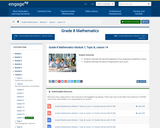Conditional Remix & Share Permitted
CC BY-NC-SA
Rating
0.0 stars

For this lesson, students calculate the decimal expansion of pi using basic properties of area. Students estimate the value of expressions such as pi2.

Subject:
Mathematics
Material Type:
Lesson Plan
Provider:
EngageNY
02/24/2017Rating
0.0 stars

This lesson unit allows students to demonstrate their understanding of classifying numbers as rational or irrational and moving between different representations of rational and irrational numbers.

Subject:
Mathematics
Material Type:
Unit of Study
Provider:
MARS, Mathematics Assessment Resource Service
03/22/2017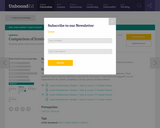Conditional Remix & Share Permitted
CC BY-NC-SA
Rating
0.0 stars

In this lesson, students place irrational numbers in their approximate locations on a number line.

Subject:
Mathematics
Material Type:
Lesson Plan
Provider:
Unbound Ed
Author:
Unbound Ed
04/23/2019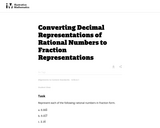Unrestricted Use
CC BY
Rating
0.0 stars

Standard 8.NS.1 requires students to "convert a decimal expansion which repeats eventually into a rational number." Despite this choice of wording, the numbers in this task are rational numbers regardless of choice of representation. For example, 0.333 and 1/3 are two different ways of representing the same number.

Subject:
Mathematics
Material Type:
Activity/Lab
Provider:
Illustrative Mathematics
Provider Set:
Illustrative Mathematics
Author:
Illustrative Mathematics
05/01/2012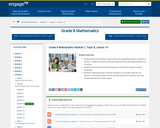Conditional Remix & Share Permitted
CC BY-NC-SA
Rating
0.0 stars

For this lesson, students know the intuitive reason why every repeating decimal is equal to a fraction. Students convert a decimal expansion that eventually repeats into a fraction. Students know that the decimal expansions of rational numbers repeat eventually. Students understand that irrational numbers are numbers that are not rational. Irrational numbers cannot be represented as a fraction and have infinite decimals that never repeat.

Subject:
Mathematics
Material Type:
Lesson Plan
Provider:
EngageNY
02/24/2017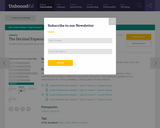Educational Use
Rating
0.0 stars

In this lesson, students use rational approximation to get the approximate decimal expansion of numbers like ?3 and ?28.

Subject:
Mathematics
Material Type:
Lesson Plan
Provider:
Unbound Ed
Author:
Unbound Ed
04/23/2019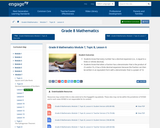Conditional Remix & Share Permitted
CC BY-NC-SA
Rating
0.0 stars

Students know that every number has a decimal expansion (i.e., is equal to a finite or infinite decimal). Students know that when a fraction has a denominator that is the product of 2’s and/or 5’s, it has a finite decimal expansion because the fraction can then be written in an equivalent form with a denominator that is a power of 10.

Subject:
Mathematics
Material Type:
Lesson Plan
Provider:
EngageNY
02/24/2017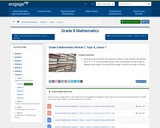Conditional Remix & Share Permitted
CC BY-NC-SA
Rating
0.0 stars

In this lesson, student will know that when the square of a side of a right triangle represented as a2, b2, c2 or is not a perfect square, they can estimate the side length as between two integers and identify the integer to which the length is closest.

Subject:
Mathematics
Material Type:
Lesson Plan
Provider:
EngageNY
02/24/2017Rating
0.0 stars

This lesson unit allows students to demonstrate their understanding of finding irrational and rational numbers to exemplify general statements and reasoning with properties of rational and irrational numbers.

Subject:
Mathematics
Material Type:
Unit of Study
Provider:
MARS, Mathematics Assessment Resource Service
03/22/2017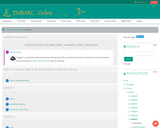Conditional Remix & Share Permitted
CC BY-NC-SA
Rating
0.0 stars

Grade 8 Module 7: Introduction to Irrational Numbers Using Geometry. Contains 23 Lessons.

Subject:
Mathematics
Material Type:
Module
Provider:
EMBARC.Online
08/13/2019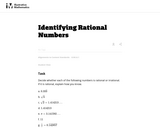Unrestricted Use
CC BY
Rating
0.0 stars

This task requires students to determine whether a number is rational or irrational. The task assumes that students are able to express a given repeating decimal as a fraction.

Subject:
Mathematics
Material Type:
Activity/Lab
Provider:
Illustrative Mathematics
Provider Set:
Illustrative Mathematics
Author:
Illustrative Mathematics
05/01/2012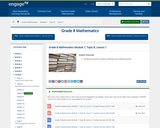Conditional Remix & Share Permitted
CC BY-NC-SA
Rating
0.0 stars

For this lesson, students know the intuitive meaning of an infinite decimal.

Subject:
Mathematics
Material Type:
Lesson Plan
Provider:
EngageNY
02/24/2017Rating
0.0 stars

Students will use candy to convert fractions to decimals and to discuss the ways in which this application might be used on a job, and what kind of jobs requires this skill.

Subject:
Mathematics
Material Type:
Activity/Lab
Lesson Plan
Provider:
Bused.org
Author:
Bused.org
02/26/2019Rating
0.0 stars

This awesome website allows elementary and middle school (K-8) to hone in on their number sense and mental math skills while competing against their classmates. It levels the math to their mastery level which is awesome.

Subject:
Mathematics
Material Type:
Activity/Lab
Author:
Kathrin Morrison1
07/30/2019Rating
0.0 stars

In this lesson, students will determine (without dividing) whether a given proper fraction will yield a repeating or terminating decimal form and will explain how the underlying principles determine the structure of the decimal form.

Subject:
Mathematics
Material Type:
Lesson Plan
Provider:
Stevens Institute of Technology
Author:
Dana T. Johnson